# AA4 – Energy and Markets

## PDAEs with Uncertainties for the Analysis, Simulation and Optimization of Energy Networks

René Henrion, Nicolas Perkowski, Caren Tischendorf

Project Members

Project Duration

01.08.2019 – 31.07.2022

Located at

HU Berlin

### Topic and Background

The project aims to support decision making for the design and control of energy networks like power networks or gas transport networks or couplings of them. Particular focus of the investigations are the uncertainties caused by fluctuations in demand and supply. Neglecting uncertainties, the transient behaviour of such networks can be described by systems of partial differential equations (e.g. the Euler equations for gas networks) coupled via algebraic constraints. A well-established approach for analyzing such systems is the formulation as an operator DAE of the form

A*(Dv(t))’ + B(v(t)) = r(t), t∈ I

with linear operators A*: Z*→ V*, D: V → Z and a nonlinear operator B: V → V*, where I is a time interval and V, Z, H are Banach spaces such that Z ⊂ H ≡ H* ⊂ Z* forms an evolution triple. To include uncertainties in the demand and supply, the function r on the right-hand side shall be modeled as a stochastic process Rt which leads to a stochastic operator DAE of the form

A*d(DVt) + B(Vt) = C(t,Vt)dRt, t∈ I.

The first challenge for the existence of solutions is the proper choice of the stochastic process Rt. Existence of solutions is not guaranteed if we allow Rt to be a Wiener process because the algebraic part needs a proper treatment. As long as the system does not contain hidden constraints, an appropriate choice for Rt would be the use of an integrated diffusion, say an integrated mean-reverting Ornstein-Uhlenbeck process, for the algebraic part and the Wiener process for the differential part. This needs a separation of both parts which can be treated by a decoupling approach.

­A second direction of research will add the optimization aspect to the consideration of PDAEs under uncertainty. The latter is assumed to be given as before by stochastic loads in the nodes of the network, however in the simpler setting of a random vector ξ (for instance, after a Karhunen-Loève expansion of the original stochastic process). The decision u to be optimized could represent a certain design variable. The aim is to find optimal decisions which are robust in a probabilistic sense with respect to later actions of the random parameter. This results in the formulation of an optimization problem subject to a probabilistic constraint:

min f(u): A*(Dv(t))’ + B(v(t)) = r(ξ, t), (t ∈ I), ℙ[g(u, v, ξ, t)≤ 0 ∀t ∈ I] ≥ p

In this model, a decision u is declared to be feasible whenever the probability of satisfying the infinite stochastic inequality system g(u, v, ξ, t) ≤ 0 ∀t ∈ I is satisfied with a probability larger than a specified level p ∈ (0, 1). The inequalities might represent, for instance, pressure bounds in a gas network. Probabilistic constraints represent a major model within stochastic optimization which is widely applied in engineering sciences, first of all in power management. Traditionally, they are considered in the framework of operations research, i.e., of finite-dimensional optimization.

### Goals and Methodologies

A first goal will be to obtain a (possibly local) solution theory for the considered equations. For that purpose we will first study monotone parabolic SPDAEs and we will extend existing work on coupled systems to the stochastic setting. By extending the analysis to “locally monotone” coefficients, we hope to treat interacting networks of Navier-Stokes equations. Networks of Euler equations could then be derived in the vanishing viscosity limit. Additionally, we aim to exploit the port-Hamiltonian structure of the network equations for the construction of proper numerical schemes. In order to address the optimization problem, we plan to start with the question of feasibility of solutions. On one hand we want to exploit ideas of the perturbation analysis that allows us to develop sufficient criteria for the validity of the inequality constraints for transient solutions. On the other hand, we intend to incorporate for the first time probabilistic constraints (moreover of implicit type) into optimization problems subject to uncertain PDAEs.

### Current Work

For our first direction of research, “Monotone SPDEs with Algebraic Constraints”, we consider coupled systems of the form

dx(t) + f(t,x,y,z,[ω])dt – R(t,x,y,z,[ω])dWtR = 0,       (SDE)

g(t,x,y,z,[ω]) = 0,        (AE)

dz(t) – A(t,x,y,z,[ω])dt – B(t,x,y,z,[ω])dWtB = 0,      (SPDE)

consisting of a stochastic differential equation (SDE), an algebraic constraint (AE) which might contain a stochastic process and a stochastic partial differential equation (SPDE). This special semi-explicit scheme empowers the decoupling approach and naturally resembles the structure arising in circuit and gas network simulation. Using this ansatz, we obtain existence and uniqueness results. Here, we have several opportunities to adapt the prototype to different settings. With similar assumptions, we may consider systems with multiple SDEs or SPDEs and even missing equations (e.g. the AE).

In the second direction of research, “Optimization Problems with Probabilistic Constraints”, we developed an optimization scheme for (currently) small gas networks, using the spheric-radial decomposition (SRD). Our numerical experiments produce in this case promising results compared to Monte Carlo (MC) and Quasi-Monte Carlo (QMC) methods.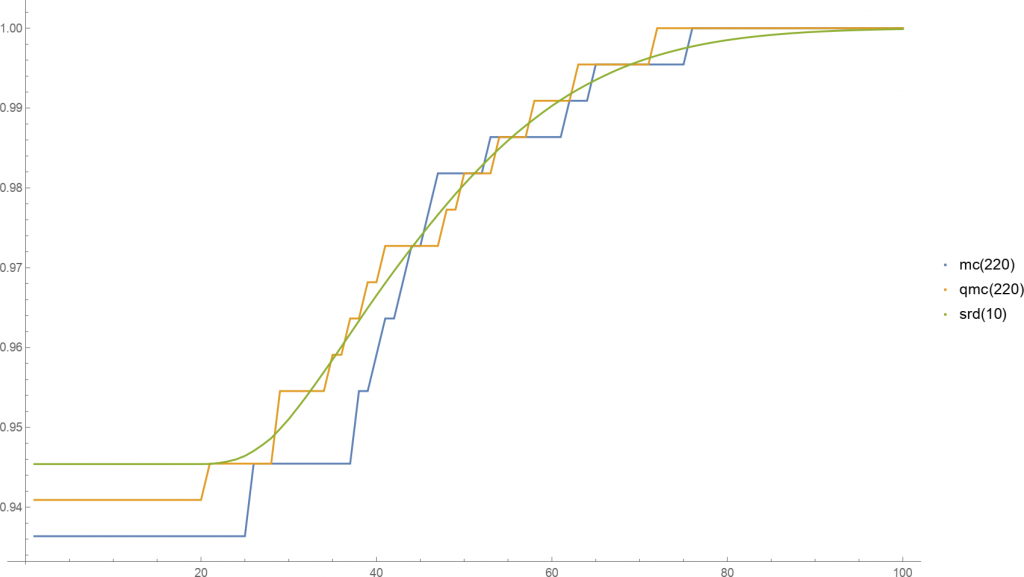Plots of the probability function for MC, QMC and SRD with respective number of samples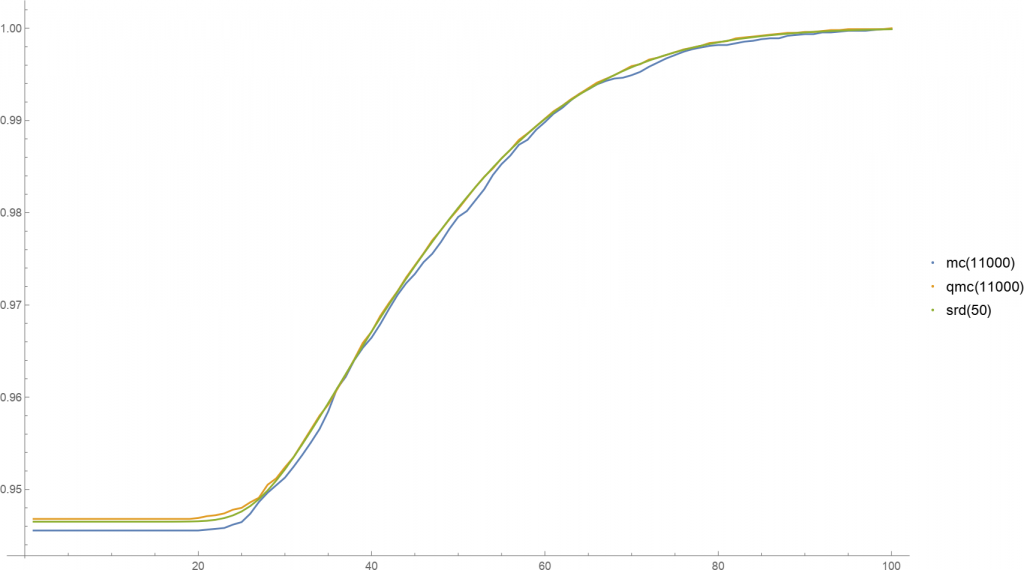Plots of the probability function for MC, QMC and SRD with respective number of samples

In contrast to the Monte Carlo and Quasi-Monte Carlo methods, the SRD method produces a smooth approximation. Accordingly, each step is more costly to compute, e.g., 10 SRD sample points compare to 220 MC or QMC samples in computation time. However, in this case the SRD method did not improve after 50 samples and MC/QMC seem to converge to this ‘limit’. This indicates that SRD produces quicker good results by having a faster convergence.

### Numerical Tests

In the first direction of research, we are currently testing the above SPDAE system in the circuit and gas network settings. For the former, we consider a simple circuit coupled with a thermally active resistor at Λr: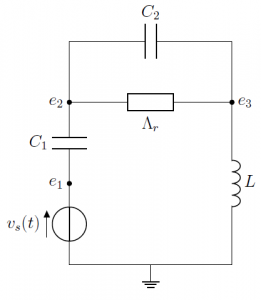Due to the semi-explicit structure of the above SPDAE prototype, we have to rearrange the circuit equations arising from the modified nodal analysis (MNA) a bit. To include stochastics into the system, we have several possibilities. The first is to simply add stochastic forces to the respective equations, whereas the second is exclusive to the SPDE. Here, we can add an stochastic process on the boundary to simulate uncertain ambient temperatures outside of the circuit area. In this case, this yields the following operatorswhere x = (e2, e3 – e2, jL), y = e1 and z = T –T . In the stochastic force B, the first part describes stochastics on the boundary and the second internally. Therefore, φ is a process as well and depends on the boundary. Finally, under some assumptions this system is uniquely solvable as this fits the prototype.

For the gas network simulation, we tested numerical schemes on the following test network with a compressor: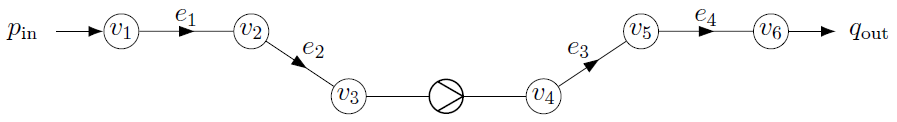We applied as stochastic algebraic constraints the pressure pin, mass flux qout and the pressure difference yc at the compressor (between v4 and v3):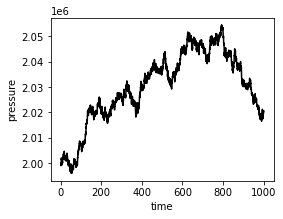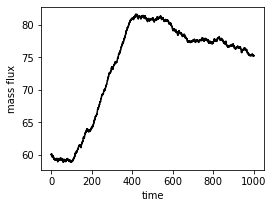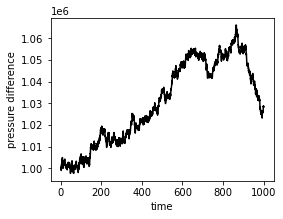In both settings, the circuit and the gas network simulation, we could verify the theoretical convergence results and got the expected convergence order 1 (for additive noise) for the drift-implicit Euler-Maruyama scheme: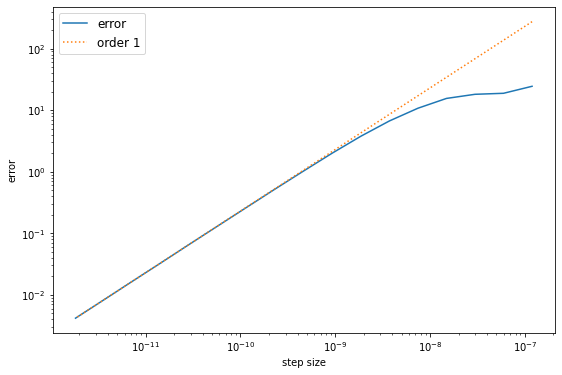Error estimate in the circuit simulation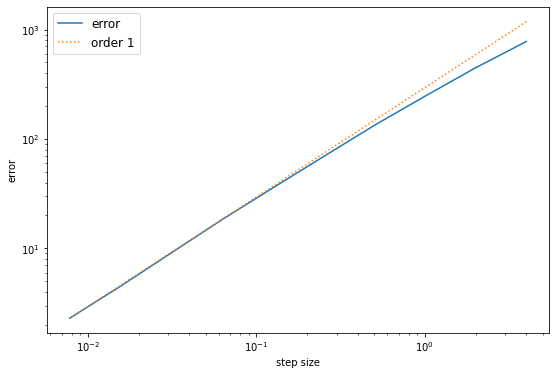Error estimate in the gas network simulation

For the second direction of research, we are currently investigating the computed optimal solutions from the SRD method. For this, we fitted an exponential model with multivariate Gaussian parameter ξ and got the following significant description of the demand

z(t,ξ) = ξ1 + ξ2 exp(-ξ3(t-ξ4)2) + ξ5 exp(-ξ3(t-ξ6)2).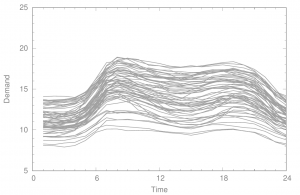Sampled historical data

Using the SRD method, we got the following free capacities.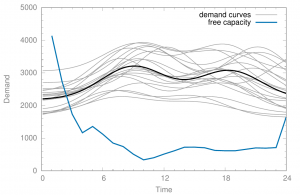Sampled demand curves and calculated free capacity

Finally when adding the computed free capacity to 20 randomly sampled demand curves, we get as expected two curves which to do not meet the pressure constraint. So, we have a 90% chance to meet the pressure constraint as desired.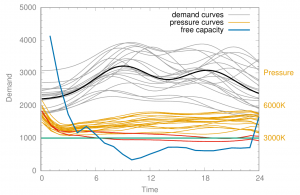Checking the pressure constraint for samples with added computed free capacity

Project Webpages

Selected Publications

• Adelhütte, D., Aßmann, D., Grandòn, T. G., Gugat, M., Heitsch, H., Henrion, R., Liers, F., Nitsche, S., Schultz, R., Stingl, M., Wintergerst, D. (2021). Joint Model of Probabilistic-Robust (Probust) Constraints Applied to Gas Network Optimization. Vietnam Journal of Mathematics, 49(4), 1097–1130. https://doi.org/10.1007/s10013-020-00434-y
• Farshbaf-Shaker, M. H., Gugat, M., Heitsch, H., Henrion, R. (2020). Optimal Neumann Boundary Control of a Vibrating String with Uncertain Initial Data and Probabilistic Terminal Constraints. SIAM Journal on Control and Optimization, 58(4), 2288–2311. https://doi.org/10.1137/19M1269944
• Cortes Garcia, I., Schöps, S., Strohm, C., Tischendorf, C. (2020). Generalized Elements for a Structural Analysis of Circuits. In: Reis T., Grundel S., Schöps S. (eds) Progress in Differential-Algebraic Equations II. Differential-Algebraic Equations Forum. Springer, Cham. https://doi.org/10.1007/978-3-030-53905-4_13
• Schade, M. (2023). Numerical Analysis and Simulation of Coupled Systems of Stochastic Partial Differential Equations with Algebraic Constraints. Ph.D. thesis. Humboldt-Universität zu Berlin. Submitted.

Selected Pictures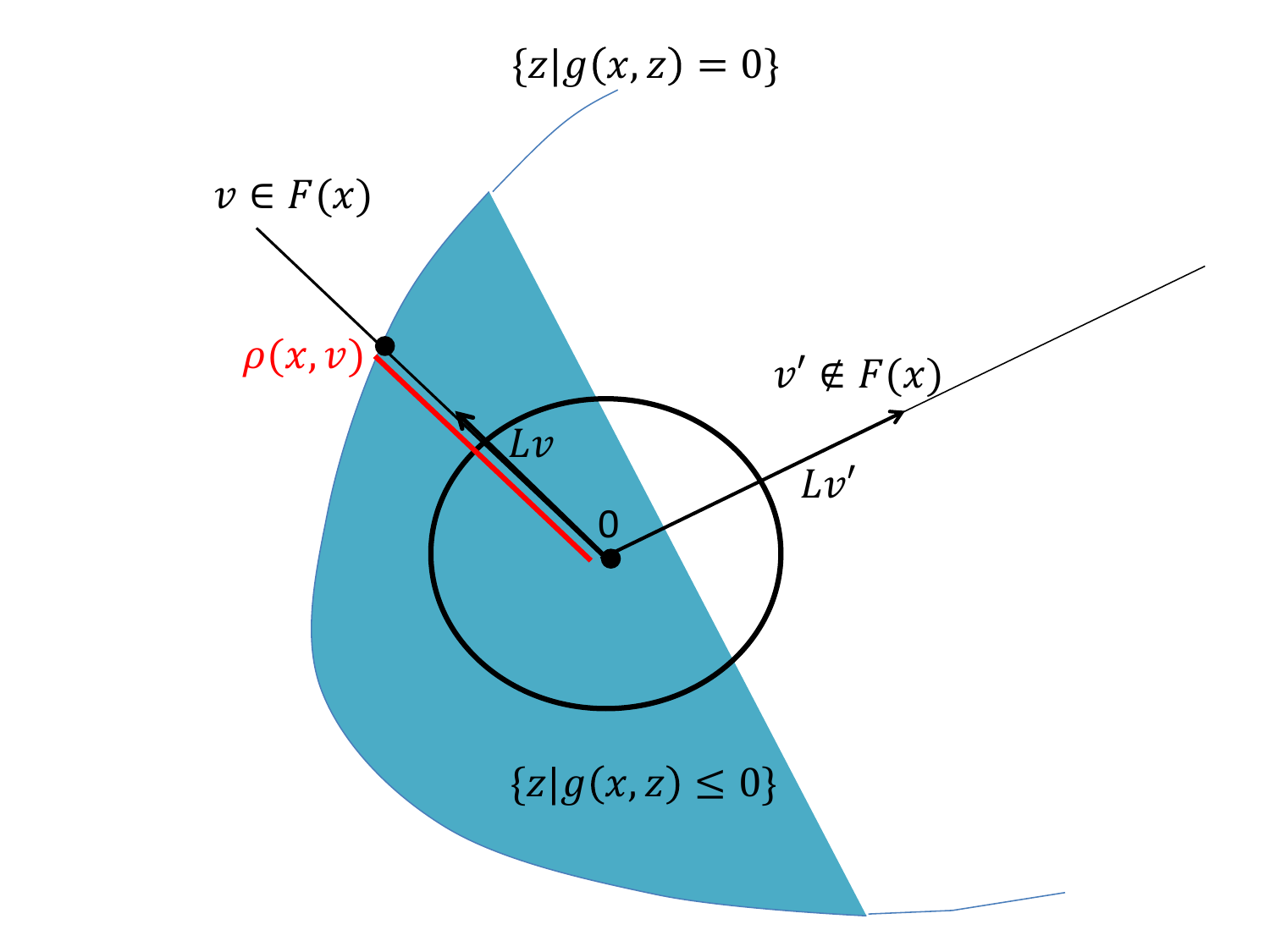Spherical-radial decomposition for the optimization problem

Please insert any kind of pictures (photos, diagramms, simulations, graphics) related to the project in the above right field (Image with Text), by choosing the green plus image on top of the text editor. (You will be directed to the media library where you can add new files.)
(We need pictures for a lot of purposes in different contexts, like posters, scientific reports, flyers, website,…
Please upload pictures that might be just nice to look at, illustrate, explain or summarize your work.)